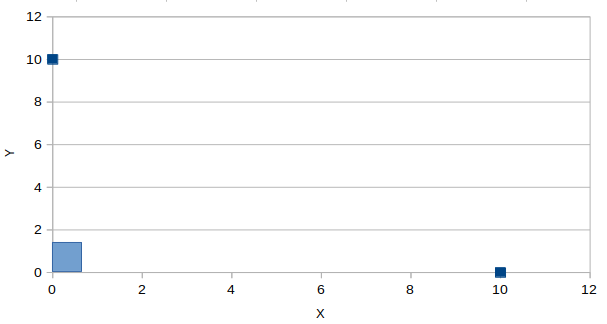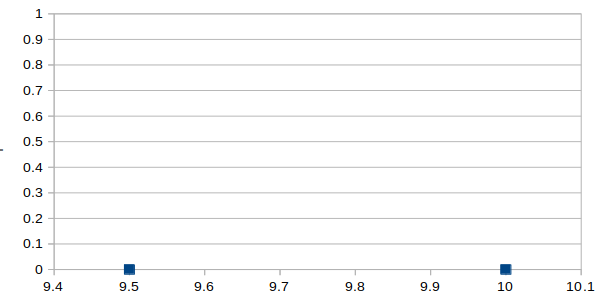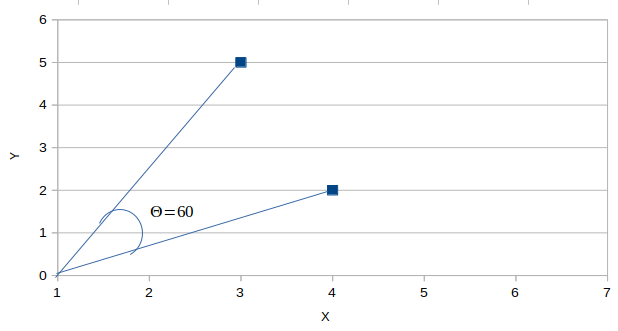# What is Cosine Distance & Cosine Similarity?

## Cosine Distance & Cosine Similarity:

Cosine distance & Cosine Similarity metric is mainly used to find similarities between two data points. As the cosine distance between the data points increases, the cosine similarity, or the amount of similarity decreases, and vice versa. Thus, Points closer to each other are more similar than points that are far away from each other. Cosine similarity is given by Cos θ, and cosine distance is 1- Cos θ. Example:-θ = 90

In the above image, there are two data points shown in blue, the angle between these points is 90 degrees, and Cos 90 = 0. Therefore, the shown two points are not similar, and their cosine distance is 1 — Cos 90 = 1.θ = 0

Now if the angle between the two points is 0 degrees in the above figure, then the cosine similarity, Cos 0 = 1 and Cosine distance is 1- Cos 0 = 0. Then we can interpret that the two points are 100% similar to each other.In the above figure, imagine the value of θ to be 60 degrees, then by cosine similarity formula, Cos 60 =0.5 and Cosine distance is 1- 0.5 = 0.5. Therefore the points are 50% similar to each other.

## Where is it used?

Cosine metric is mainly used in Collaborative Filtering based recommendation systems to offer future recommendations to users.

Taking the example of a movie recommendation system, Suppose one user (User #1) has watched movies like The Fault in our Stars, and The Notebook, which are of romantic genres, and another user (User #2) has watched movies like The Proposal, and Notting Hill, which are also of romantic genres. So the recommendation system will use this data to recommend User #1 to see The Proposal, and Notting Hill as User #1 and User #2 both prefer the romantic genre and its likely that User #1 will like to watch another romantic genre movie and not a horror one.

Similarly, Suppose User #1 loves to watch movies based on horror, and User #2 loves the romance genre. In this case, User #2 won’t be suggested to watch a horror movie as there is no similarity between the romantic genre and the horror genre.